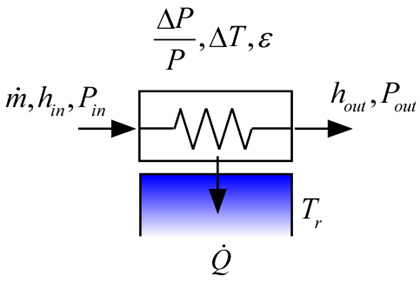Contents - index

HeatExchanger1_CLThe procedure HeatExchanger1_CL models a heat exchanger in which a fluid is interacting with a reservoir (i.e., a source or sink that has infinite capacitance).  The approach temperature difference is specified.  This function works with real fluids, ideal gas, incompressible, or brines.

Inputs:

F\$:  fluid string identifier

C:  Concentration (%) - note that this is only necessary if the fluid is a brine; otherwise set C=0

m_dot:  mass flow rate (kg/s or lbm/hr)

h_in:  inlet specific enthalpy (J/kg, kJ/kg, or Btu/lbm)

P_in:  inlet pressure (bar, atm, Pa, kPa, MPa)

T_r:  temperature of the reservoir (K, C, R, or F)

DT:  approach temperature difference (K, C, R, or F)

DPoverP: pressure drop normalized by absolute pressure (-)

Outputs:

h_out:  outlet specific enthalpy (J/kg, kJ/kg, or Btu/lbm)

P_out:  outlet pressure (bar, atm, Pa, kPa, MPa)

Q_dot:  heat transfer rate (W, kW or Btu/hr)

into device if T_r>T_in

out of device if T_r<T_in

eff:  effectiveness (-)

Example 1:

\$UnitSystem SI Mass J K Pa

F\$='DowTherm_Q'

C=20 [%]

m_dot=0.01 [kg/s]

T_in=380 [K]

P_in=4e6 [Pa]

h_in=enthalpy(F\$,T=T_in,P=P_in)

T_r=300 [K]

DT=10 [K]

DPoverP=0.01 [-]

Call heatexchanger1_cl(F\$, 0, m_dot, h_in, P_in, T_r, DT, DPoverP: h_out, P_out, Q_dot, eff)

{Solution:

h_out = 125990 J/kg

P_out = 3.96-0e6 Pa

Q_dot = 1274 W

eff = 0.8827}

Example 2:

\$UnitSystem SI Mass J K Pa

F\$='EG'

C=20 [%]

m_dot=0.01 [kg/s]

T_in=380 [K]

P_in=4e6 [Pa]

h_in=enthalpy(F\$, T=T_in, C=C, P=P_in)

T_r=300 [K]

DT=10 [K]

DPoverP=0.01 [-]

Call heatexchanger1_cl(F\$, C, m_dot, h_in, P_in, T_r, DT, DPoverP: h_out, P_out, Q_dot, eff)

{Solution:

h_out = 119770 J/kg

P_out = 3.960e6 Pa

Q_dot = 2793 W

eff = 0.8770}